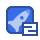# 移动渐近线法98收藏

This function mmasub performs one MMA-iteration, aimed at % solving the nonlinear programming problem: % % Minimize f_0(x) + a_0*z + sum( c_i*y_i + 0.5*d_i*(y_i)^2 ) % subject to f_i(x) - a_i*z - y_i <= 0, i = 1,...,m % xmin_j <= x_j = 0, y_i >= 0, i = 1,...,m %*** INPUT: % % m = The number of general constraints. % n = The number of variables x_j. % iter = Current iteration number ( =1 the first time mmasub is called). % xval = Column vector with the current values of the variables x_j. % xmin = Column vector with the lower bounds for the variables x_j. % xmax = Column vector with the upper bounds for the variables x_j. % xold1 = xval, one iteration ago (provided that iter>1). % xold2 = xval, two iterations ago (provided that iter>2). % f0val = The value of the objective function f_0 at xval. % df0dx = Column vector with the derivatives of the objective function % f_0 with respect to the variables x_j, calculated at xval. % df0dx2 = Column vector with the non-mixed second derivatives of the % objective function f_0 with respect to the variables x_j, % calculated at xval. df0dx2(j) = the second derivative % of f_0 with respect to x_j (twice). % Important note: If second derivatives are not available, % simply let df0dx2 = 0*df0dx. % fval = Column vector with the values of the constraint functions f_i, % calculated at xval. % dfdx = (m x n)-matrix with the derivatives of the constraint functions % f_i with respect to the variables x_j, calculated at xval. % dfdx(i,j) = the derivative of f_i with respect to x_j. % dfdx2 = (m x n)-matrix with the non-mixed second derivatives of the % constraint functions f_i with respect to the variables x_j, % calculated at xval. dfdx2(i,j) = the second derivative % of f_i with respect to x_j (twice). %

...展开详情

• 1
资源
• 0
粉丝
•等级移动渐近线法 47积分/C币 立即下载
1/0